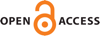Download this articleFor screen For printingRecent IssuesThe Journal About the Journal Editorial Board Editorial Interests Subscriptions Submission Guidelines Submission Page Policies for Authors Ethics Statement ISSN (electronic): 1472-2739 ISSN (print): 1472-2747 Author Index To Appear Other MSP JournalsConstraints on families of smooth $4$–manifolds from $\mathrm{Pin}^{-}(2)$–monopole

### Hokuto Konno and Nobuhiro Nakamura

Algebraic & Geometric Topology 23 (2023) 419–438##### Abstract

Using the Seiberg–Witten monopole equations, Baraglia recently proved that the inclusion $\mathrm{Diff}\left(X\right)↪\mathrm{Homeo}\left(X\right)$ is not a weak homotopy equivalence for most of simply connected closed smooth $4$–manifolds $X$. We generalize Baraglia’s result by using the ${\mathrm{Pin}}^{-}\left(2\right)$–monopole equations instead. We also give new examples of $4$–manifolds $X$ for which ${\pi }_{0}\left(\mathrm{Diff}\left(X\right)\right)\to {\pi }_{0}\left(\mathrm{Homeo}\left(X\right)\right)$ are not surjections.

##### Keywords
diffeomorphism, homeomorphism, 4–manifold
Primary: 57R57
Secondary: 57S05
##### Publication